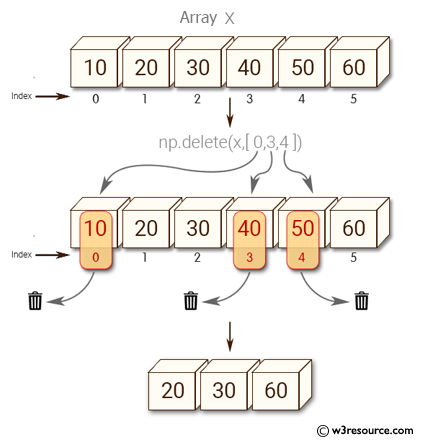﻿ NumPy: Remove specific elements in a NumPy array - w3resource# NumPy: Remove specific elements in a NumPy array

## NumPy: Array Object Exercise-89 with Solution

Write a NumPy program to remove specific elements in a NumPy array.

Pictorial Presentation:Sample Solution:

Python Code:

``````import numpy as np
x = np.array([10, 20, 30, 40, 50, 60, 70, 80, 90, 100])
index = [0, 3, 4]
print("Original array:")
print(x)
print("Delete first, fourth and fifth elements:")
new_x = np.delete(x, index)
print(new_x)
```
```

Sample Output:

```Original array:
[ 10  20  30  40  50  60  70  80  90 100]
Delete first, fourth and fifth elements:
[ 20  30  60  70  80  90 100]
```

Python Code Editor:

Have another way to solve this solution? Contribute your code (and comments) through Disqus.

What is the difficulty level of this exercise?

Test your Python skills with w3resource's quiz

﻿

## Python: Tips of the Day

Set comprehension:

```>>> m = {x ** 2 for x in range(5)}
>>> m
{0, 1, 4, 9, 16}
```